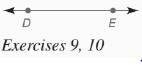Chapter 7.CR, Problem 9CRElementary Geometry For College St...

7th Edition
Alexander + 2 others
ISBN: 9781337614085

Solutions

Chapter
SectionElementary Geometry For College St...

7th Edition
Alexander + 2 others
ISBN: 9781337614085
Textbook Problem

In Review Exercises 7 to 13, sketch and describe the locus in a plane.Find the locus of points equidistant from points D and E .To determine

To find: The locus of points that are equidistant from points D and E.

Explanation

Given:

The line with points D and E is shown below,

Definition used:

A locus is the set of all points and only those points that satisfy a given condition (or set of conditions).

Calculation:

Consider the given figure.

The locus is the line that is the perpendicular bisector of DE¯<

Still sussing out bartleby?

Check out a sample textbook solution.

See a sample solution

The Solution to Your Study Problems

Bartleby provides explanations to thousands of textbook problems written by our experts, many with advanced degrees!

Get Started

Find more solutions based on key concepts